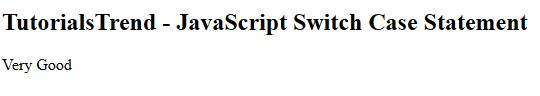﻿ JavaScript Conditional Statement - TutorialsTrend
This Angular tutorial helps you get started with Angular quickly and effectively through many practical examples.
`

# JavaScript Conditional Statement

Conditional Statements in JavaScript are used to make decisions based on the conditions. These conditions are specified by a set of conditional statements having boolean expressions which are evaluated to a boolean value true or false. This process is called decision making.

Everyone has experienced the situation where they can only receive their favorite cycle if they pass their exam with more than 90% of the marks. If you don't, you'll be penalised.  The result you get is based on the condition of whether or not you get 90% marks. These are similar to how conditional statements work.

There are several conditional statements in JavaScript that you can use to make decisions:

1. The if statement
2. The if else statement
3. The if else if else statement
4. The switch case statement

The Pictorial representation of Conditional Statement as following: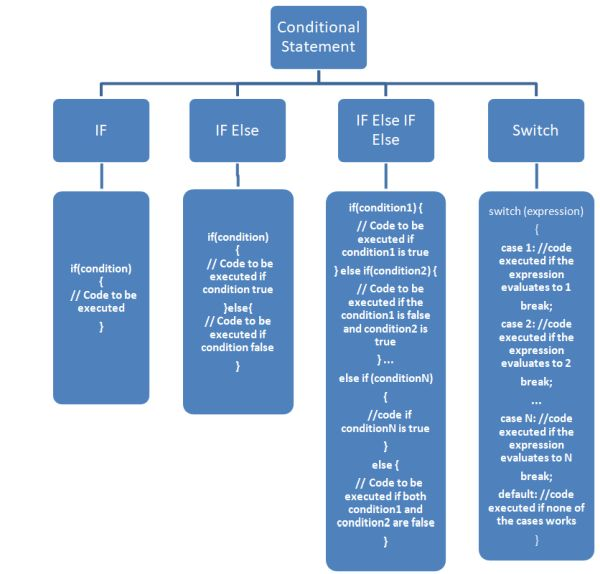1. The if statement

The JavaScript keyword if alerts the interpreter that a conditional statement is about to be used. A block of code is only execute using the if statement if the given condition is true.

Flowchart of IF Statement

Following is the execution flow diagram of IF statement.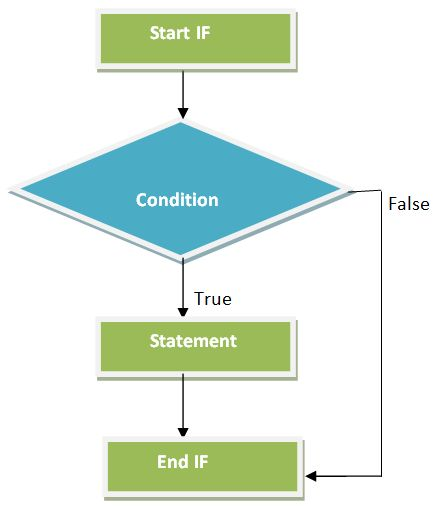The syntax for if statement is like this.

``````if(condition) {
// Code to be executed
}``````

Example

The following code defines the if Statement in JavaScript.

``````<!DOCTYPE html>
<html lang="en">
<meta charset="utf-8">
<title></title>
<body>
<h2>TutorialsTrend - JavaScript if Statement</h2>
<script>
var percentage = 50
if (percentage >= 40) {
}
</script>
</body>
</html>``````

Output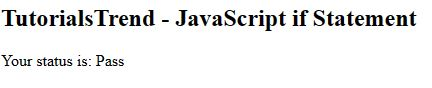2. The if else Statement

you can improve the decision-making capabilities of your JavaScript program. By offering an alternate option by including an else statement in the if statement, In The if else statement, if the condition is true then the statements inside the if block is executed and if not, the else block is executed.

Flowchart of IF Else Statement

Following is the execution flow diagram of IF Else statement.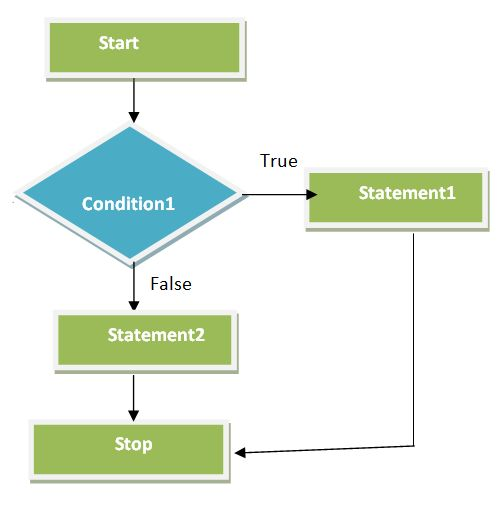The syntax for if else statement is like this.

``````if(condition) {
// Code to be executed if condition is true
} else {
// Code to be executed if condition is false
}``````

Example

The following code defines the if else Statement in JavaScript.

``````<!DOCTYPE html>
<html lang="en">
<meta charset="utf-8">
<title></title>
<body>
<h2>TutorialsTrend - JavaScript if else Statement</h2>
<script>
var percentage = 50
if (percentage >= 33) {
}else{
}
</script>
</body>
</html>``````

Output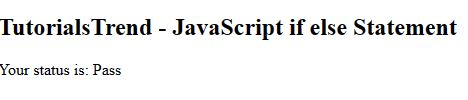3. The if else if else Statement

Multiple if else statements can be combined using the if else if else special statement. The JavaScript interpreter will check the if condition first. If it is true, the if block runs; if false, it will move onto the else if condition. This checks if the condition is true for the second condition but false for the first one. This process continues for all the else if statements. If none of the conditions is true, the code in the else block is run.

Flowchart of IF Else IF Else Statement

Following is the execution flow diagram of IF Else IF Else statement.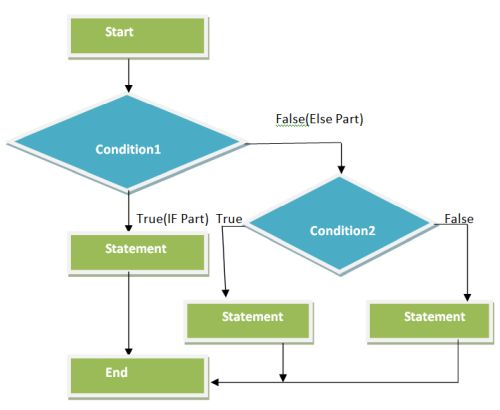The syntax for if else if else statement is like this.

``````if(condition1) {
// Code to be executed if condition1 is true
} else if(condition2) {
// Code to be executed if the condition1 is false and condition2 is true
} ...
else if (conditionN)
{
//code if conditionN is true
}
else {
// Code to be executed if both condition1 and condition2 are false
}``````

Example

The following code defines the if else if else Statement in JavaScript.

``````<!DOCTYPE html>
<html lang="en">
<meta charset="utf-8">
<title></title>
<body>
<h2>TutorialsTrend - JavaScript if else if else Statement</h2>
<script>
var percentage =70
if (percentage >= 90) {
}else if (percentage >= 80) {
}else if (percentage >= 70) {
}else if (percentage >= 60) {
}else if (percentage >= 50) {
}else{
}
</script>
</body>
</html>``````

Output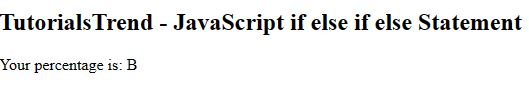4. The switch case statement

A switch statement allows a variable to be tested for equality against a list of values. Each value is called a case, and the variable being switched on is checked for each switch case. When a break statement is reached, the switch terminates, and the flow of control jumps to the next line following the switch statement.  The default case can be used for performing a task when none of the cases is true. No break is needed in the default case.

Flowchart of Switch Statement

Following is the execution flow diagram of Switch statement.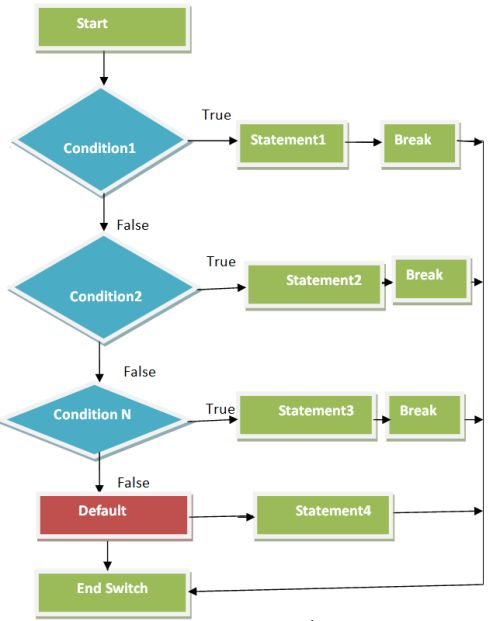The syntax for switch statement is like this.

``````switch (expression)
{
case 1: //code executed if the expression evaluates to 1
break;
case 2: //code executed if the expression evaluates to 2
break;
...
case N: //code executed if the expression evaluates to N
break;
default: //code executed if none of the cases works
}``````

Example

The following code defines the switch Statement in JavaScript.

``````<!DOCTYPE html>
<html lang="en">
<meta charset="utf-8">
<title>TutorialsTrend - JavaScript Switch Case Statement</title>
<body>
<h2>TutorialsTrend - JavaScript Switch Case Statement</h2>
<script>
case 'A' :
document.write("Excellent");
break;
case 'B' :
document.write("Very Good");
break;
case 'C' :
document.write("Good");
break;
case 'D' :
document.write("Try again");
break;
default :